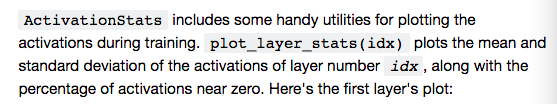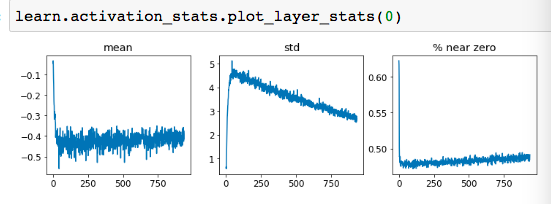# Lesson 13 graph axes

On Lesson 13 Convolutions, there are a number of graphs that I would like to know what are the axes used:

Questions:

1. In the graphs below, is the x-axis the layer number, with zero being the first layer and 750 the output layer?1. In the graphs below, what does the x-axis represent?My knowledge is still patchy, and clarifying things like these will help solidify it.

Thank you!

Maria

The X axis are the mini-batch number.

There are N (=60,000 for MNIST) / bs (=64 or 512 for this example) number of mini-batches or number of times the model is utilized for each epoch
So the total range of X = (Total number of Epochs) * (N / bs or number of mini-batches)

For the top graph, bs = 64: (1) * (60,000 / 64) = 937
For the bottom graph, bs = 512: (1) * (60,000 / 512) = 117

@meanpenguin I see now, thanks again!Maria